Easy

# Basic Statistics for Data Science Beginners

Written by
Editors
Updated on
May 10, 2023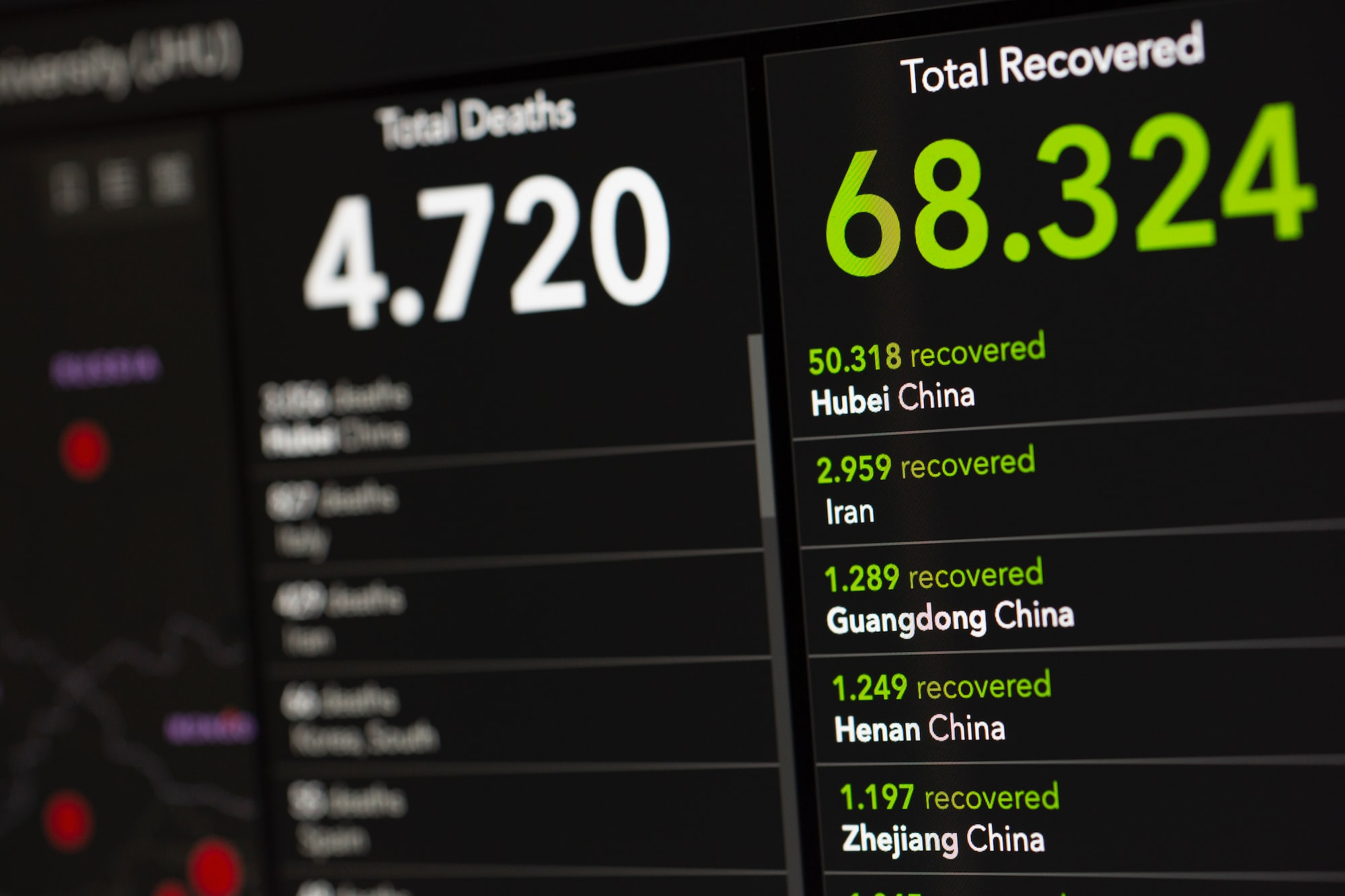Oops! Something went wrong while submitting the form.

Statistics is a necessary tool for data scientists to gather and analyze large amounts of data to develop insights.

As a data scientist, your role will require sound statistical skills to make sense of quantitative data to spot trends and make predictions. However, most learners are not very clear about what to learn or where to start.

Statistics is invariably required from the beginning of data processing to the end at every single step.

In this article, we will provide clarity about the key Statistical concepts required for effective data science and also suggest resources according to your background.

Learning these concepts will help you build the statistical intuition and cognitive skills you need for Data Science and Machine Learning.

## Overview

Data Science involves Descriptive Statistics and Inferential Statistics, and competency in both will lead you to a rewarding data career.

If you are eager to learn statistics, first understand the difference between the two major categories within statistics.

Descriptive Statistics: It is hard to review, summarize, and communicate a lot of raw information, but descriptive statistics, allows to present the data purposefully.

Descriptive statistics provide analyses and descriptions of the data, and a way to visualize the data.

Inferential Statistics: Inferential statistics helps to reach conclusions and draw inferences through mathematical calculations.

Inferential Statistics allow us to infer trends and make predictions about a population based on a study of the data.

As the name suggests, Descriptive Statistics describes the population and on the other end, Inferential Statistics is used to make the generalisation about the population based on the samples.

These methods are critical to advancements across scientific fields like Data Science and Machine Learning.

Let's take a closer look at the key concepts imperative for Data Science.

# Basic Statistics Concepts for Data Science

To become a data scientist, you need the mathematical intuition and statistical reasoning for computational techniques that are useful in data analysis.

In simple words, you must build the statistical fluency by learning to use key statistical formulas to illustrate and communicate analytical results.

You must become equipped with fundamental concepts of descriptive statistics and probability theory, which include the key concepts of probability distribution, statistical significance, hypothesis testing, and regression.

Bayesian thinking is also necessary for data science and it's very important to learn its concepts required for machine learning like conditional probability, priors and posteriors, and maximum likelihood.

The ideal student for learning these concepts is ready to master the next level in programming, i.e. Statistical programming with Python, R or Julia.

You can learn these concepts from the notable data science educators:

### 1. Descriptive Statistics

The Descriptive Statistics enable us to present raw data constructively and summarize data points in a practical way.

You must learn these basic concepts of research methods to perform simple statistical analysis, visualize data, predict future trends from the data, etc.

### 1.1 Normal Distribution

Normal distribution is one of the most common probability distribution function used for many types of real-world applications.

For instance, variables like people's heights, weights and IQ follow normal distribution closely.

In a normal distribution, we represent data samples in a plot.

The Normal distribution looks like this symmetric bell looking thing, which is why it's often called a bell curve. It is also known as the Gaussian Curve.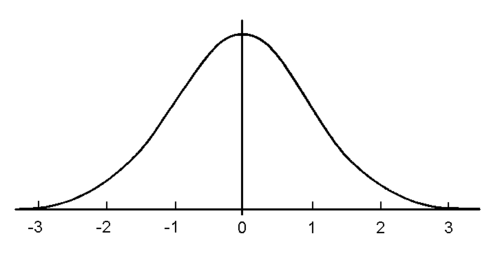This bell curve is centered around its mean and spreads out with decreasing probability as you move away in either direction.

68–95–99.7 rule

For a normal distribution between plus + and minus -1 one standard deviation, there is 68% of the distributions data.

Between plus + or minus -2 standard deviations there is 95% of the data.

Finally, between plus or minus 3 standard deviations, there is 99.7% of the data.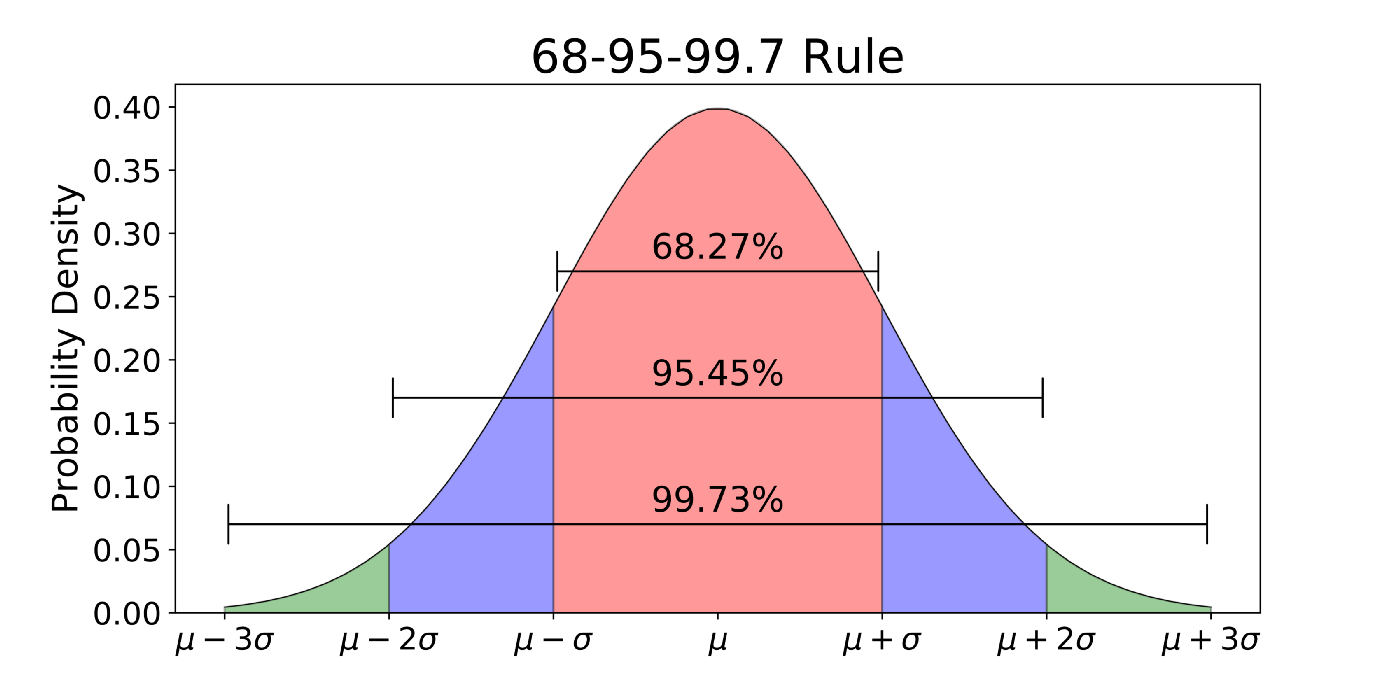Normal distribution is widely applicable, and each application comes with its own mean, standard deviation, and units.

This distribution function applies in most ML Algorithms and you must learn the concept of the Normal Distribution in a detailed manner because the Linear Models perform well if the data is normally distributed. You will also need it for Central Limit Theorem and Exploratory Data Analysis.

### 1.2 Central Tendency

Using a central tendency, we identify the central point of the data. The three important parts of central tendency are Mean, Median and Mode.

Mean: The arithmetic average of all the observations (values) in the dataset.

Median: The middle value of the data arranged from the least to the greatest.

Mode: The most frequent value in the dataset. Some datasets can have over one mode, meaning multiple values that occur most frequently. If there are two values with the highest frequency, then we have a bimodal distribution. If there are over two modes, then we have a multi-modal distribution.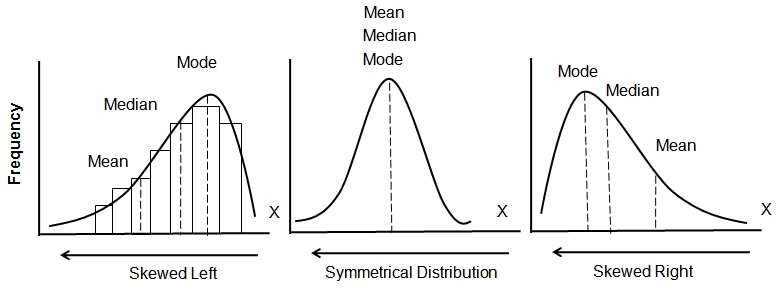It is very easy to learn Mean Median and Mode, including Skewness & Kurtosis.

### 1.3 Skewness

It is a measure of symmetry, but sometimes the distribution does not exhibit any form of symmetry.

We can see visually what happens to the measures of central tendency when we encounter asymmetrical distribution.

Notice how these measures spread when the normal distribution (Symmetrical) is distorted. When data accumulates on the left side, it is positive skew and when data accumulates on the right side; we have a negative skew.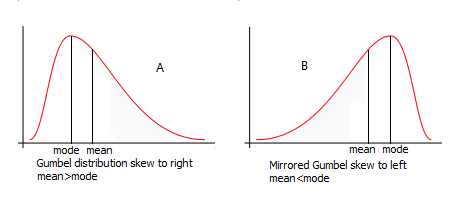Learn to detect the extent of asymmetry

### 1.4 Kurtosis

It is a measure of whether the data is heavy-tailed or light-tailed relative to a normal distribution.

The distributions with a large kurtosis have tails that are larger than normal distributions whereas, negative kurtosis will have smaller tails than normal distributions.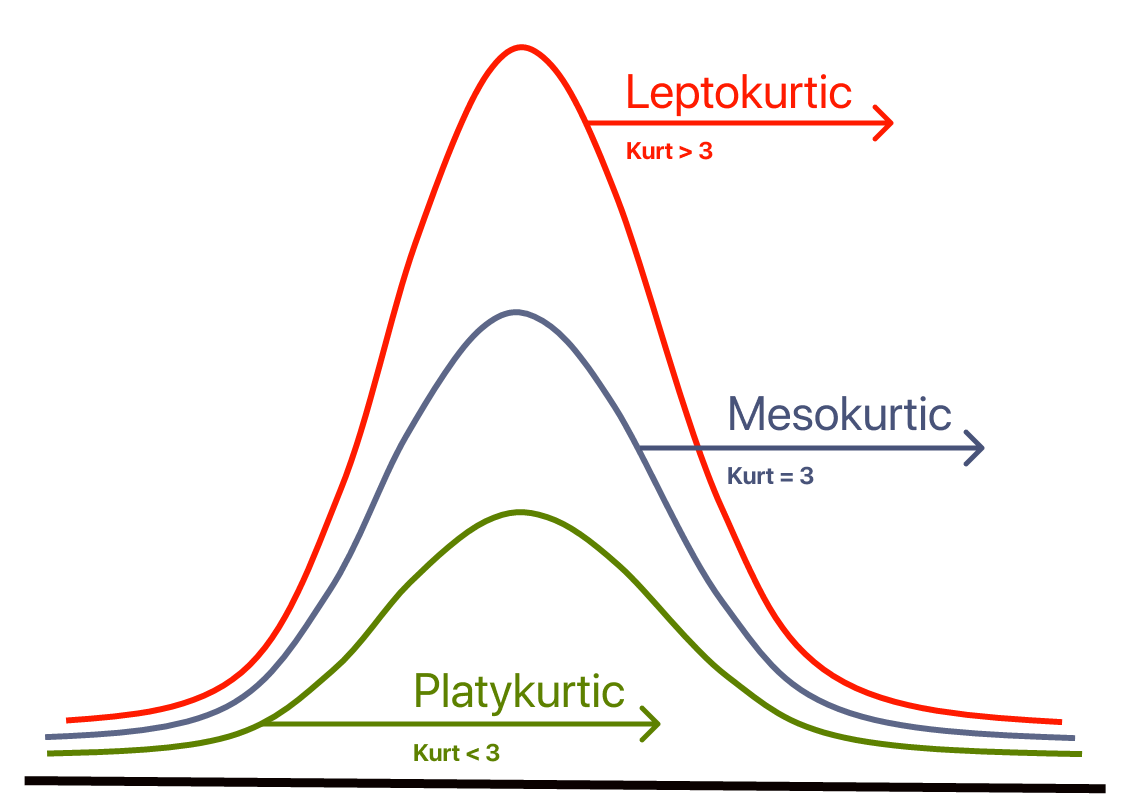This is caused by the variability in the distribution.

• Lepto-kurtic – It is a curve having a higher peak than normal curve because of more concentration of the items near the center.
• Platy-kurtic: It is a curve having a lower peak and is flatter than the normal peak because of less concentration of the items near the center.
• Meso-Kurtic: It is a curve having a normal peak or normal curve. When there is equal distribution around the center value (mean), in that event mean, median, and mode are equal.
Learning the distribution of data is a very important aspect

### 1.5 Variability

Variability measures the distance between the data-point from the central mean of the distribution.

Variability is usually defined in terms of distance

• How far apart scores are from each other
• How far apart scores are from the mean
• How representative a score is of the data set as a whole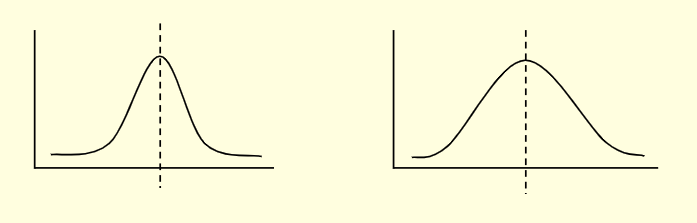The measures of variability include range, variance, standard-deviation, and inter-quartile ranges.

Range: It is the simplest way of describing variability. The range only considers the two extreme scores and ignores any values in between.

Standard Deviation: This measure expresses the variability in terms of a typical deviation in the data set. It is commonly used to learn whether a specific data point is standard and expected, or unusual and unexpected.

• Low standard deviation tells us that the data is closely clustered around the mean or average
• High standard deviation shows that the data is dispersed over a wider range of values, us that the data is closely clustered around the mean or average
Standard deviation is used when the distribution of data is approximately normal, resembling a bell curve.

Variance: The variance is a measure of variability. It is essentially the average of the squared differences from the mean.

Variance is very important for data analysis and you must learn all the concepts thoroughly.

Percentiles, Quartiles, and Interquartile Range (IQR):

Percentiles: Percentiles tell us how a value compares to other values. A percentile is a value below which a certain percentage of observations lie.

Quantiles: A quantile determines how many values in a distribution are above or below a certain limit. It can also refer to dividing a probability distribution into areas of equal probability.

Interquartile Range (IQR): The interquartile range measures the spread of the middle half of data. It is the range for the middle 50% of data. We use IQR to assess the variability where most of the values lie.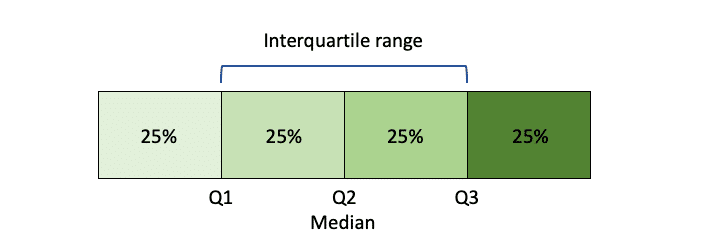These concepts may sound intimidating, but are simple to learn.

### 2. Inferential Statistics

Inferential Statistics provide the bases for predictions, forecasts, and estimates that are used to transform information into knowledge.

The process of “inferring” insights or concluding from the data through probability is called “Inferential Statistics.”

Probability distributions, hypothesis testing, correlation testing and regression analysis fall under the category of inferential statistics.

The best real-world example of “

Inferential Statistics

” is predicting the relationship between death and smoking habit.

Inferential Statistics comprise generalizing from samples to population, hypothesis testing, and making predictions.

Some of these techniques are helpful for data science.

### 2.1 Central Limit Theorem

Central Limit Theorem is one of the most fundamental and a simple concept in statistics.

It is essentially the sampling distribution of the mean.

As the sample size increases, the distribution of the mean of sample values is normal.

The mean of the smaller sample data is the same as that of the mean of the larger population. So, the resulting standard deviation is also equal to the standard deviation of the population.

It is easier to understand CTL if you are familiar with the Normal distribution.

The CTL applies to nearly all types of probability distributions. These can range from normal, left-skewed, right-skewed, and uniform.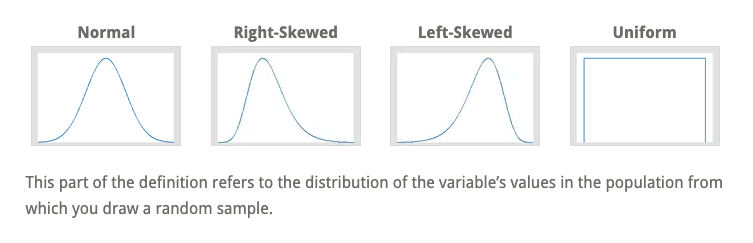Understand and practice CTL concepts with

• Normal Population
• Dichotomous Outcome
• Skewed Distribution

The central limit theorem is important for statistics because of the normality assumption and the precision of the estimates.

Learn concepts like estimation of the population mean, the laws of frequency of errors, including how to calculate margin error with the z-score of the percentage of confidence level.

### 2.2 Hypothesis Testing

Hypothesis testing is the heart of all statistics that allows us to make inferences about the world.

It is the measure of assumption, an educated guess about the world around us.

Hypothesis testing, in simple words, is a process whereby a Statistical Analyst investigates an assumption regarding a population parameter. The methods employed depend on the data used and the reason for the analysis.

Normally, the methods include estimating population properties like mean, differences between means, proportions, and the relations between variables.

It is most often used by Data Scientists to prove specific predictions, called hypotheses, that arise from theories.

Companies often use this technique to implement new features on their websites or mobile applications.

There are two hypotheses that we require to test against each other:

Both are mutually exclusive, and only one of the two hypotheses will always be true.

Null Hypothesis: It is a prediction of no relationship between the variables and may state that the population mean return is equal to zero.

Alternate Hypothesis: It is the initial hypothesis that predicts a relationship between variables. The alternative hypothesis is completely opposite of a null hypothesis.

It's not always clear how the null and alternative hypothesis should be formulated and, for this reason, the context of the situation is important in determining how the hypotheses should be stated.

In data science, the applications of hypothesis testing are analytical and involve an attempt to gather evidence to support a research hypothesis.

Correct hypothesis formulation takes a lot of practice. Understand the terminology, testing process and concepts well enough to make inferences with real-world examples.

### 2.3 ANOVA

Analysis of variance—ANOVA test, in its simplest form, is used to observe whether the differences between groups of data are statistically important.

The ANOVA test applies when there are over two independent groups. Using ANOVA, we test our hypothesis to identify if there is a need to reject the null hypothesis or accept the alternate hypothesis.

ANOVA performs testing with a minimal error rate to see if there’s a difference between multiple groups.

Cases when you might want to test multiple groups:

• A group of cancer patients are trying three different therapies: Chemotherapy, Hormone Therapy and Immunotherapy. You want to study if one therapy is stronger than the others.
• A company makes two affordable smartphones using two different operating systems. They want to learn if one is better than the other.
These techniques are used in Data Science and Machine Learning to decide the result of the dataset.

You will need to apply statistical knowledge to make a confident and reliable decision as a Data Scientist.

Understand the concepts involved in learning how to solve problems with ANOVA test; learn One-Way ANOVA, Two-Way ANOVA, and N-Way ANOVA, including its formulas.

### 2.4 Regression Analysis

Regression is a form of qualitative data analysis to find trends in data.

It is a predictive modeling technique that helps companies to understand what their data points represent and uses them skillfully with other analytical techniques to make better decisions.

Regression analysis is the “go-to method in analytics,” says Tom Redman.

In Data Science, the evaluation of the relation between the variables is called Regression Analysis, and these variables refer to the properties or characteristics of certain events or objects.

We need to grasp the following terms:

• Dependent Variable: This is the key factor that you’re seeking to understand or predict.
• Independent Variables: These are the factors or elements that you hypothesize have an impact on the dependent variable.

Essentially, regression analysis helps to make the best guess at using a set of data to make predictions.

There is simple regression analysis and multiple (multi-variable) regression analysis.

Simple regression: There is only one predictor variable, a single x variable for each dependent “y” variable: (x1, Y1).

Multiple regression: This analysis uses multiple “x” variables for each independent variable: (x1)1, (x2)1, (x3)1, Y1).

If the function is non-linear, then we have a non-linear regression.

Regression allows data crunching to provide answers for the important business questions: Which aspects matter most? Which can we ignore? How do those elements interact with each other? How certain are we about these considerations?

The regression method of forecasting is very helpful for analyzing the data in the following ways:

• Predicting sales
• Assuming demand and supply
• Understanding inventory levels
• Analyze how variables impact all these factors

For Data Science and Machine Learning, we use Python libraries like NumPy, Pylab, and Scikit-learn for Simple and multiple regression analysis.

A thorough understanding of regression and multi-variable regression would serve as a good foundation for learning machine learning algorithms such as logistic regression, K-nearest neighbor, and support vector machine.

## Best Resources to Data Science Learners

The aspiring Data Scientists must know these necessary statistics concepts and possess skills for data analysis, data visualization, machine learning, etc.

We have compiled the high-quality learning resources suitable according to the experience-level. Along with courses, we very much recommend a few books to cement your knowledge.

### Conclusion

We went through Statistical requirements for Data Science. Competency in both descriptive statistics and inferential statistics will equip you for statistical programming.

Learning Statistics is not very difficult. In fact, it will bootstrap your cognitive abilities and help improve your programming skills.

Also, we hope the learning resources (recommendations) help you expand your data science knowledge and fight your fear of discovering what’s happening behind the scenes.

Credits: Banner Image by Markus Spiske / Unsplash

## Trending Stacks

Please bear with us, an update is coming soon.
Data Engineering
Master Data Engineering with these powerful tools, techniques, and resources.
Data Science
Discover a wealth of data science resources to elevate your analytical skills.
Cloud Computing
Elevate your cloud computing skills with up-to-date resources.